# Excel Formulas for Open Channel Flow Measurement Calculations with Parshall Flume Equations

## Background

The Parshall flume is an open channel flow measurement device that is well suited for flows containing suspended solids because it tends to be self-flushing. Parshall flumes also have less head loss than weirs for open channel flow measurement. Using the Excel formulas in the spreadsheets that can be downloaded from links later in this article, the flow rate through a Parshall flume can be calculated for both free flow and submerged flow conditions, in either U.S. units or S.I. units. For more background about the Parshall flume for open channel flow measurement and specifications for its dimensions, see the article, "Open Channel Flow Measurement 2: the Parshall Flume."

## Free Flow and Submerged Flow Through Parshall Flumes

When the flow through a Parshall flume is unaffected by flow conditions downstream of the flume, there is said to be "free flow" through the flume. A hydraulic jump will be visible in the throat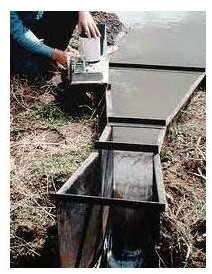of the Parshall flume during free flow conditions. When the downstream flow "backs up" into the flume, obscuring the hydraulic jump, the flow is said to be submerged. Good practice calls for design of Parshall flumes to operate under free flow conditions because the flow rate can be measured more accurately and only one head measurement is needed. In the past, however, it was common to design Parshall flumes for 90% submergence, so there are still many flumes operating for open channel flow measurement under submerged conditions.

Image Credit: Montezuma Valley Irrigation Company

The conditions for free flow through a Parshall flume are specified in the form of a maximum submergence ratio for each of several ranges of throat widths. The submergence ratio, S, is defined as Hb/Ha, where Ha is the head measurement needed for free flow calculations, measured at a point 2/3 of the length of the straight-sided converging section upstream from the beginning of the Parshall flume throat, and Hb is the head measured at the throat. The introductory Parshall flume article mentioned above has diagrams and specifications showing the specified locations in the Parshall flume for measurement of Ha and Hb.

A summary of the conditions required for free flow through a Parshall flume are as follows:

• for 1" < W < 3": free flow exists for Hb/Ha < 0.5
• for 6" < W < 9": free flow exists for Hb/Ha < 0.6
• for 1' < W < 8': free flow exists for Hb/Ha < 0.7
• for 10' < W < 50': free flow exists for Hb/Ha < 0.8

If the submergence ratio is greater than the values given above for any given throat width, then the flow through the Parshall flume is submerged, and both of the head measurements, Ha and Hb, are needed for the Parshall flume equations and calculations.

Within this article there are Excel spreadsheets that can be downloaded to calculate the flow rate through a Parshall flume for both free flow and submerged conditions with either U.S. or S.I units.

## Excel Formulas for Free Flow Parshall Flume Calculations

The Parshall flume equation for free flow conditions is Qfree = C Han, where

• Qfree is the open channel flow rate through the Parshall flume under free flow conditions, in cfs
• Ha is the head measured at the correct point in the converging section of the Parshall flume as described in the previous section, in ft
• C and n are constants for a given Parshall flume throat width, W.

The image at the left gives the constants C and n in the Parshall flume equations for standard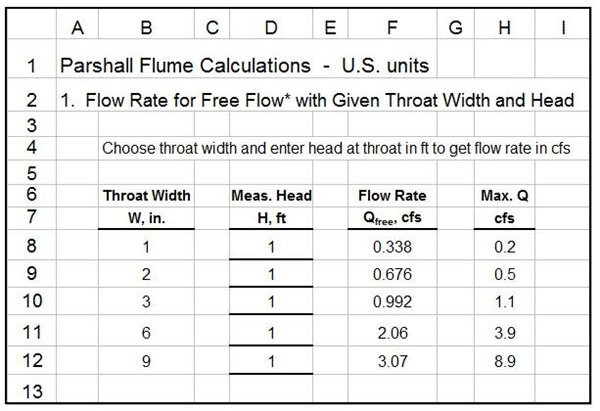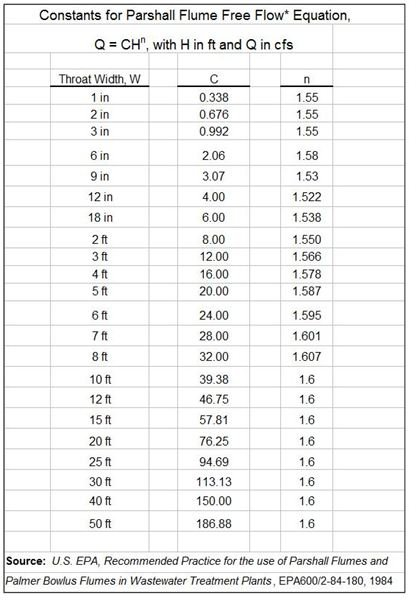throat widths ranging from one inch to fifty feet. The image at the right shows an Excel spreadsheet for calculating the flow rate through a Parshall flume under free flow conditions. The user simply needs to enter the measured head, Ha, in the row for the correct flume throat width. Then the Excel formulas will calculate the flow rate for free flow through the flume, Qfree. The image only shows throat widths from 1" to 9", but the spreadsheet that can be downloaded through the link below has throat widths up to 50′.

The last column in the spreadsheet shows a maximum recommended flow rate for each throat width. This can be used to determine what throat width should be used for a given Parshall flume application.

The calculated flow rate will be correct only if the flow through the Parshall flume is indeed free flow, that is with a submergence ratio less than the maximum specified in the list in the previous section. If the submergence ratio is greater than the allowable value for free flow, then the methods of the next section must be used to calculate the flow rate under submerged conditions.

## Excel Formulas for Submerged Parshall Flume Calculations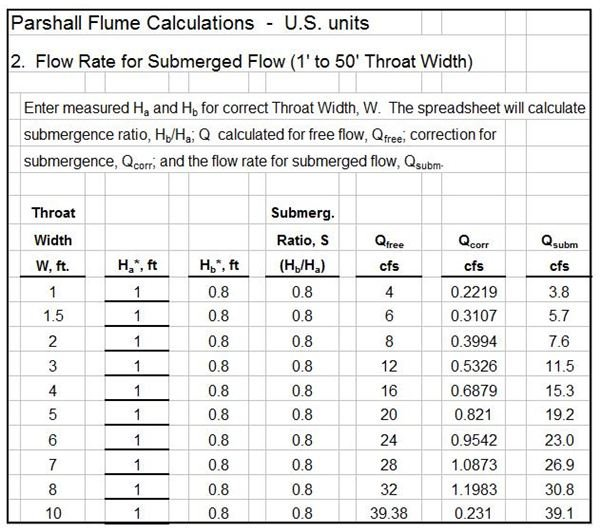An image of an Excel spreadsheet to calculate flow rate through a Parshall flume under submerged flow conditions is shown at the left. This image shows throat widths from 1′ to 10′, but the Excel spreadsheets that can be downloaded through the links below cover throat widths from 1′ to 50′. The Parshall flume equations for submerged flow, as used by the Excel formulas in the spreadsheets, are as follows:

Qsubm = QfreeQcorr, where

• Qsubm is the flow rate through the Parshall flume for a submerged flow condition, in cfs
• Qfree is the flow rate calculated with the equation, Qfree = C Han, as described in the previous section, in cfs
• Qcorr is a flow correction factor calculated from the equations below for the correct throat width, W

For 10′ < W < 50′ : Qcorr = (W/10)(3.364 + 20.19 S2 ln S)2, where: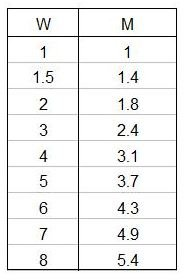• W = throat width in ft
• S = submergence ratio = Hb/Ha

For 1′ < W < 8′ : Qcorr = 0.000132 M Ha2.123 e9.284S, where M is a constant for each throat width in the range from 1′ to 8′ as shown in the image at the right. Ha and S are as defined above.

The use of this spreadsheet requires input of measured values for Ha and Hb. Then the Excel formulas calculate the submergence ratio, S; Qfree, Qcorr, and Qsubm, using the Parshall flume equations discussed above.

## References

References for Further Information:

1. U.S. EPA, Recommended Practice for the Use of Parshall Flume and Palmer Bowlus Flumes in Wastewater Treatment plants, EPA600/2-84-180, 1984

2. Wahl, Tony L., Equations for Computing Submerged Flow in Parshall Flumes, Bureau of Reclamation, Denver, Colorado, USA

3. U.S. Dept. of the Interior, Bureau of Reclamation, Water Measurement Manual, 2001 revised, 1997 third edition

## This post is part of the series: Excel Spreadsheets for Open Channel Flow Measurement Calculations

These contain Excel spreadsheets that can be downloaded for open channel flow measurement devices, such as V-notch weirs and rectangular weirs. The Excel spreadsheets can be used as a weir flow calculated in each case.